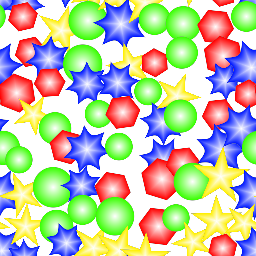# Tiler node¶

The Tiler node tiles several instances of its input with optional scale and rotation variations. Overlapping instances are mixed with each other using a lighten filter.

The Tiler also has a color version whose input is in RGBA format.## Inputs¶

The Tiler node accepts two inputs:

• The Source inputs is the image to be splat into the output.

• The Mask input is a greyscale image that is used as a mask and affects each instance’s value.

## Outputs¶

The Tiler node outputs the splat image and a secondary output that assigns a random color to each tile.

The greyscale tiler has a third output that assigns a UV layout to each tile.

## Parameters¶

The Tiler node accepts the following parameters:

• Tile X and Tile Y, the number of columns and rows of of the tile pattern.

• Overlap is the number of overlapping instances for each instance.

• Inputs is the number of alternate shapes in the input (1, 4 or 16). Images containing several shapes can easily be created using the Tile2x2 node.

• Scale X and Scale Y are the scale along X and Y axes applied to each instance.

• Fixed Offset is an offset applied to all odd lines.

• Offset is the maximum random offset applied to each instance (relative to tiles size).

• RndRotate is the maximum angle of the random rotation applied to each instance.

• RndScale is the amount of random scaling applied to each instance.

• RndValue is the amount of random value applied to each instance.

• Variations: if checked, the node will tile different variations of its input (i.e. roll a different seed for each instance)

## Example images¶